# NEETprep Bank NEET Physics Systems of Particles and Rotational Motion Questions Solved

A block of mass m moving at a velocity v collides with another block of mass 2m at rest. The lighter block comes to rest after collision. Find the coefficient of restitution.

(a) $\frac{1}{2}$                                               (b) $1$

(c) $\frac{1}{3}$                                               (d) $\frac{1}{4}$

From conservation of linear momentum we can see that velocity of 2m will become $\frac{\mathrm{v}}{2}$ after collision (as mass is doubled)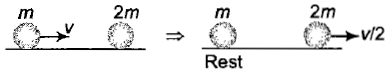Difficulty Level:

• 50%
• 29%
• 14%
• 9%
PMT - 2011

A small mass attached to a string rotates on a frictionless table top as shown. If the tension on the string is increased by pulling the string causing the radius of the circular motion to decrease by a factor of 2, the kinetic energy of the mass will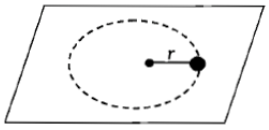(a) Increase by a factor of 4                                       (b) Decrease by a factor of 2

(c) Remain constant                                                   (d) Increase by a factor of 2

Difficulty Level:

• 36%
• 30%
• 26%
• 10%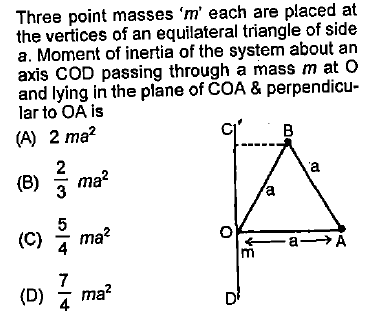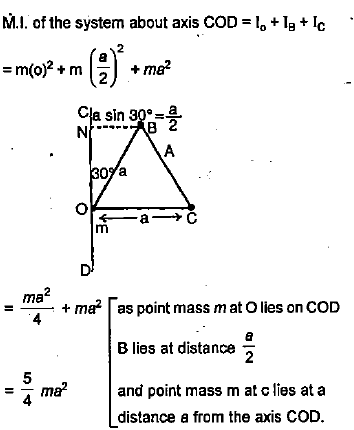Difficulty Level:

• 16%
• 28%
• 48%
• 10%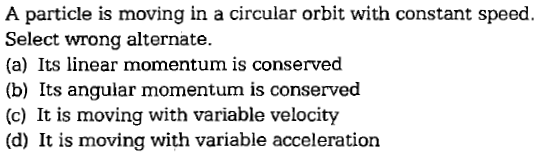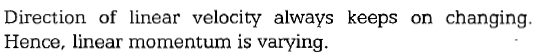Difficulty Level:

• 35%
• 31%
• 18%
• 18%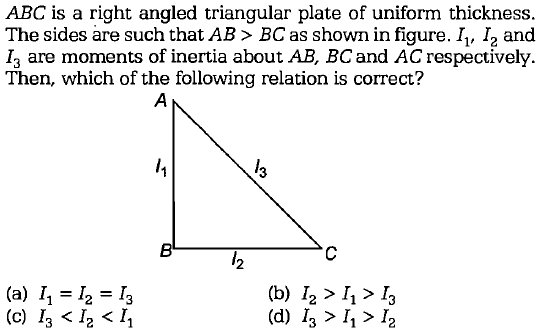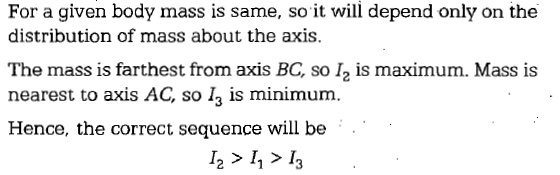Difficulty Level:

• 19%
• 43%
• 20%
• 21%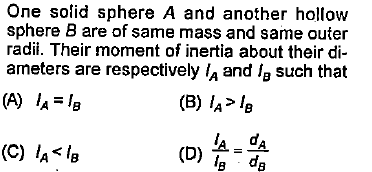Difficulty Level:

• 9%
• 22%
• 64%
• 7%
PMT - 1997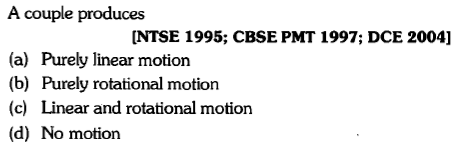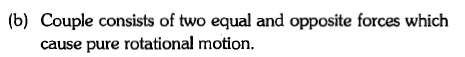Difficulty Level:

• 7%
• 55%
• 33%
• 7%

A particle of mass 1 kg is kept at (1m, 1m, 1m). The moment of inertia of this particle about z-axis would be

1.

2.

3.

4.  None of these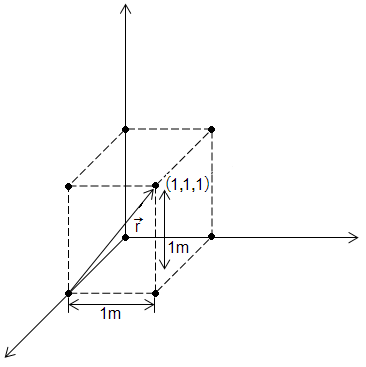Difficulty Level:

• 43%
• 39%
• 12%
• 8%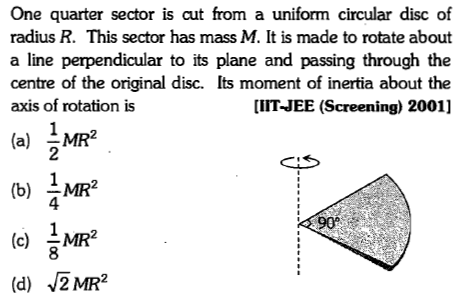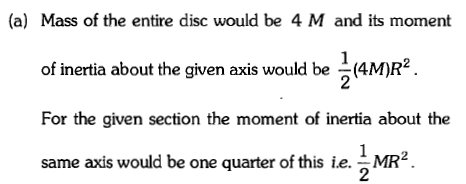Difficulty Level:

• 48%
• 25%
• 24%
• 5%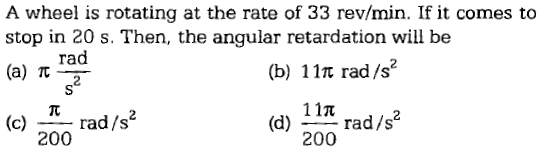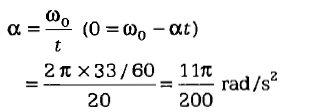Difficulty Level:

• 6%
• 15%
• 17%
• 65%